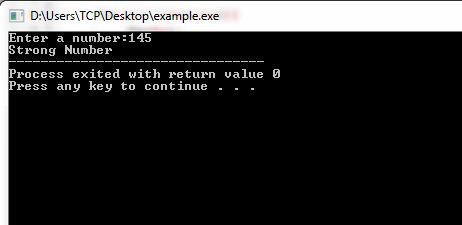# Strong Number in C

Here you will get program for strong number in C.

What is Strong Number?

A number in which the sum of factorial of individual digits is equal to the number is called strong number.

For example, 145 is a strong number because 145=(!1)+(!4)+(!5)=1+24+120=145

The below C program will check whether a given number in strong number or not.

## Program for Strong Number in C

OutputComment below if you are facing any difficulty to understand above strong number in C program.

### 5 thoughts on “Strong Number in C”

1.ZAFAR ALI KHAN

thanks
useful for students

in Program for Strong Number in C

sum=0;

is required

2.can you add python code too

3.#include

int factorial(int number)
{
int i,fact=1;

for(i=1;i<=number;i++)
{
fact=fact*i;
}

return fact;
}

int main()
{
int number,digit,sum=0,temp;

printf("Enter a number:");
scanf("%d",&number);

temp=number;

while(temp!=0)
{
digit=temp%10;
digit = factorial(digit);
sum=sum+digit;
temp=temp/10;
}

if(sum==number)
{
printf("Strong Number");
}
else
{
printf("Not Strong Number");
}

return 0;
}

4.thank u

5.Sir, This program is not working for number = 10 (10 is a strong number), so what is the actual correct program then. Will you please inform me….